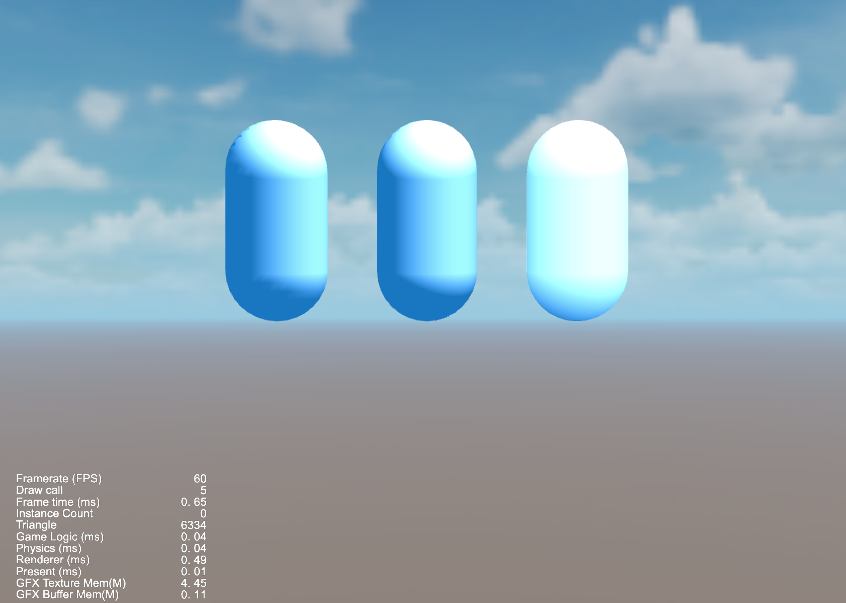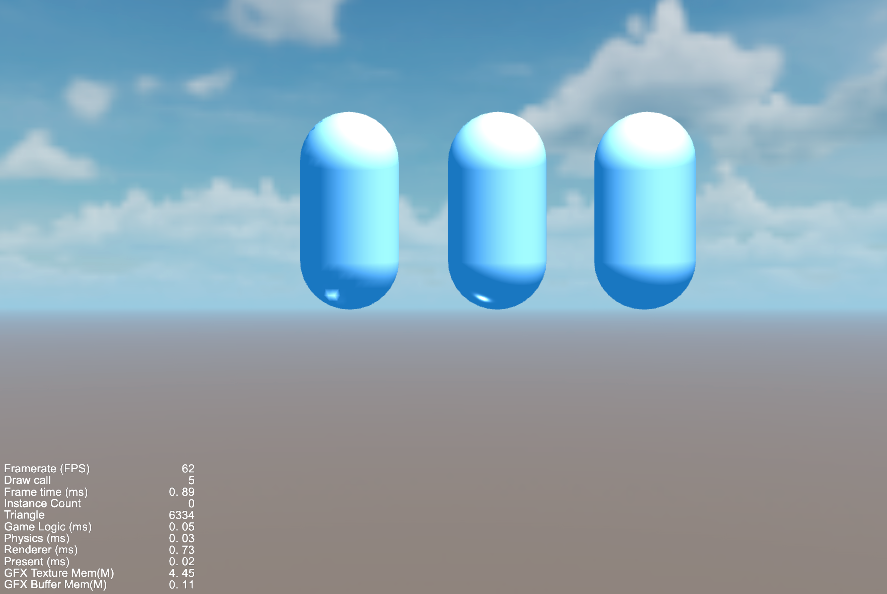• Cocos

## 二 漫反射

漫反射是光线与表面粗糙度较高的物体表面相互作用时发生的反射，这是最基本、最常见的一种反射现象。在漫反射下，光线以均匀分布的方式反射，即物体将光线向所有方向发射出去。

$C{diffuse} = (C{light} M_{diffuse} ) max(0, n \cdot l)$

### 2.1 逐顶点光照

Cocos Shader中声明一个属性 diffuseCol，初始值为白色，代表材质的漫反射颜色。

properties:
diffuseCol: { value: [1, 1, 1, 1], editor: { type: color } }

#### 2.1.1 顶点着色器

vec4 vert () {
vec4 pos = vec4(a_position, 1.0);
pos = cc_matProj * (cc_matView * cc_matWorld) * pos;

vec3 ambient = cc_ambientSky.xyz;
vec3 worldNormal = normalize((cc_matWorldIT * vec4(a_normal, 0.0)).xyz);
vec3 worldLightDir = normalize(cc_mainLitDir.xyz);
float satu = clamp(dot(worldNormal, worldLightDir), 0.0, 1.0);
vec3 diffuse = cc_mainLitColor.rgb * diffuseCol.rgb * satu;
vec4 color = vec4(ambient + diffuse, 1.0);

v_uv0 = a_texCoord;
v_color = color;
return pos;
}

#### 2.1.2 片元着色器

vec4 frag () {
vec4 o = vec4(1, 1, 1, 1);
o *= v_color;
return o;
}

### 2.2 逐像素光照

#### 2.2.1 顶点着色器

vec4 vert () {
vec4 pos = vec4(a_position, 1.0);
pos = cc_matProj * (cc_matView * cc_matWorld) * pos;

v_normal = (cc_matWorldIT * vec4(a_normal, 0.0)).xyz;

v_uv0 = a_texCoord;
v_color = a_color;
return pos;
}

#### 2.2.2 片元着色器

vec4 frag () {
vec4 o = vec4(1, 1, 1, 1);

vec3 ambient = cc_ambientSky.xyz;
vec3 worldNormal = normalize(v_normal);
vec3 worldLight = normalize(cc_mainLitDir.xyz);
float satu = clamp(dot(worldNormal, worldLight), 0.0, 1.0);
vec3 diffuse = cc_mainLitColor.rgb * diffuseCol.rgb * satu;
vec4 color = vec4(ambient + diffuse, 1.0);

o = color;
return o;
}

### 2.3 半兰伯特光照模型

$C{diffuse} = (C{light} M_{diffuse} ) ( \alpha (n \cdot l) + \beta)$

$C{diffuse} = (C{light} M_{diffuse} ) ( 0.5 (n \cdot l) + 0.5)$

vec4 frag () {
....

float halfLambert = dot(worldNormal, worldLight) * 0.5 + 0.5;
vec3 diffuse = cc_mainLitColor.rgb * diffuseCol.rgb * halfLambert;
vec4 color = vec4(ambient + diffuse, 1.0);

col = color;
return col;
}## 三 高光反射

$C{specular} = (C{light} M_{specular} ) max(0, v \cdot r)^m$

### 3.1 逐顶点光照

properties:
diffuseCol: { value: [1, 1, 1, 1], editor: { type: color } }
specularCol: { value: [1, 1, 1, 1], editor: { type: color } }
glossVal: { value: 20, editor: { range: [8, 256] } }

vec4 vert () {
vec4 pos = vec4(a_position, 1.0);
pos = cc_matProj * (cc_matView * cc_matWorld) * pos;

vec3 ambient = cc_ambientSky.xyz;
vec3 worldNormal = normalize((cc_matWorldIT * vec4(a_normal, 0.0)).xyz);
vec3 worldLightDir = normalize(cc_mainLitDir.xyz);
float satu1 = clamp(dot(worldNormal, worldLightDir), 0.0, 1.0);
vec3 diffuse = cc_mainLitColor.rgb * diffuseCol.rgb * satu1;

vec3 reflectDir = normalize(reflect(-worldLightDir, worldNormal));
vec3 viewDir = normalize(cc_cameraPos.xyz - (cc_matWorld * vec4(a_position, 1.0)).xyz);
float satu2 = clamp(dot(reflectDir, viewDir), 0.0, 1.0);
vec3 specular = cc_mainLitColor.rgb * specularCol.rgb * pow(satu2, glossVal);

vec4 color = vec4(ambient + diffuse + specular, 1.0) ;

v_uv0 = a_texCoord;
v_color = color;
return pos;
}

vec4 frag () {
vec4 o = vec4(1, 1, 1, 1);
o *= v_color;
return o;
}

### 3.2 逐像素光照

vec4 vert () {
vec4 pos = vec4(a_position, 1.0);
pos = cc_matProj * (cc_matView * cc_matWorld) * pos;

v_worldNormal = (cc_matWorldIT * vec4(a_normal, 0.0)).xyz;
v_worldPos = (cc_matWorld * vec4(a_position, 1.0)).xyz;

v_uv0 = a_texCoord;
v_color = a_color;
return pos;
}

vec4 frag () {
vec4 o = vec4(1, 1, 1, 1);

vec3 ambient = cc_ambientSky.xyz;

vec3 worldNormal = normalize(v_worldNormal);
vec3 worldLightDir = normalize(cc_mainLitDir.xyz);

float satu1 = clamp(dot(worldNormal, worldLightDir), 0.0, 1.0);
vec3 diffuse = cc_mainLitColor.rgb * diffuseCol.rgb * satu1;

vec3 reflectDir = normalize(reflect(-worldLightDir, worldNormal));
vec3 viewDir = normalize(cc_cameraPos.xyz - v_worldPos);
float satu2 = clamp(dot(reflectDir, viewDir), 0.0, 1.0);
vec3 specular = cc_mainLitColor.rgb * specularCol.rgb * pow(satu2, glossVal);

vec4 color = vec4(ambient + diffuse + specular, 1.0) ;

o = color;
return o;
}

### 3.3 Blinn-Phong光照模型

$C{specular} = (C{light} M_{specular} ) max(0, n \cdot h)^m$

vec4 frag () {
...
vec3 viewDir = normalize(cc_cameraPos.xyz - v_worldPos);
vec3 halfDir = normalize(worldLightDir + viewDir);
float satu2 = max(0.0, dot(worldNormal, halfDir));
vec3 specular = cc_mainLitColor.rgb * specularCol.rgb * pow(satu2, glossVal);

vec4 color = vec4(ambient + diffuse + specular, 1.0) ;
o = color;
return o;
}## 四 获取源码